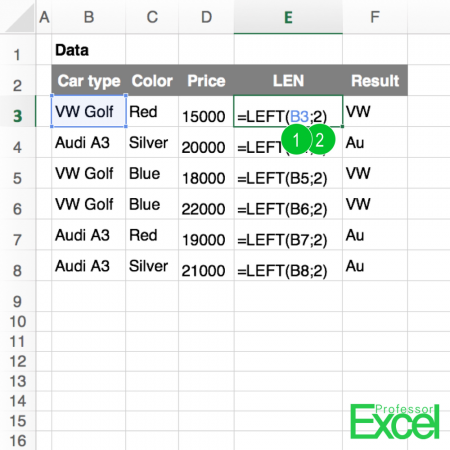# LEFT or RIGHT: Extract Text from Cells in ExcelYou got a text cell and want to separate it into two parts: The left hand side of it and the right hand side. Therefore, you should take a look at the LEFT() and RIGHT() formulas, which you can use them when you want to extract some parts of a text. LEFT() returns a number of characters of a cell, starting from the left hand side. RIGHT() does exactly the same, but starts from the right hand side.

## How to apply the LEFT() and RIGHT() formulas

The application of the two formulas is simple, as the formula only has two parts (the numbers are corresponding to the picture above):

1. The cell you want to get a specific number of characters, either from the left or right hand side. In our case it’s cell B3.
2. The number of characters you want to get returned. In our example we want to get 2 characters from the left hand side.

The complete formula above is:

`=LEFT(B3,2)`

So, if you only want to get some text from the left or right hand side of a cell, it is pretty easy to use the formula. It usually gets more complicated, when you want to have some text from the middle. In such case, you combine the two formulas.

Also, you might want to use these formulas together with the LEN() formula. The LEN() formula returns the length of a cell. Please refer to this article if you want to use them together.Henrik Schiffner is a freelance business consultant and software developer. He lives and works in Hamburg, Germany. Besides being an Excel enthusiast he loves photography and sports.